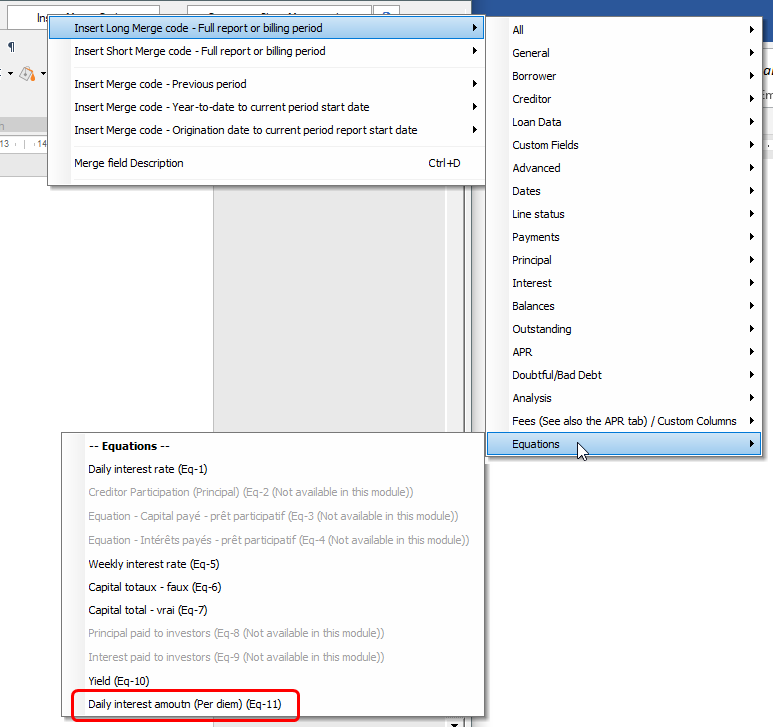## How can I create a schedule where I can see the interest that accrues on a daily basis, every day?

Q: My law firm must calculate the interest from June 30, 2019 to May 28, 2020 on an amount due by an insurance company. I must be able to see the interest that accrues every day.

Interest rate is 4% annually and amount is 150,250.33.

A: In Margill Law Edition, you would usually use the “Interest on one amount between two dates” calculation: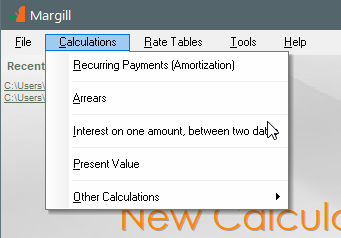Data entry: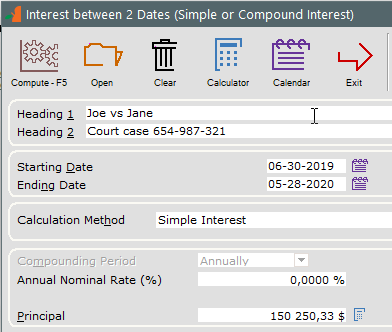This would give you the amount as two lines with a split on December 31 at midnight: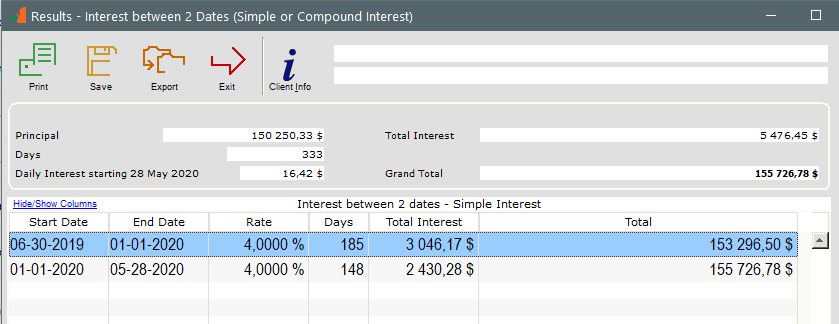I know, you want detail, lots of it, on a daily basis so instead of using “Interest on one amount between two dates”, use the very powerful “Recurring Payments (Amortization)” calculation that can do just about anything, not only loans or mortgages.

Here is how I would enter the data to see the payments every single day. Notice:

• “First Payment Date” is one date after my “Origination Date” or start date
• “Payment Method” = “Payments set to 0.00”.
• For “Number of Payments”, I right clicked with the mouse to enter 05-28-2020 and Margill calculates a cool 333 payments (of 0.00)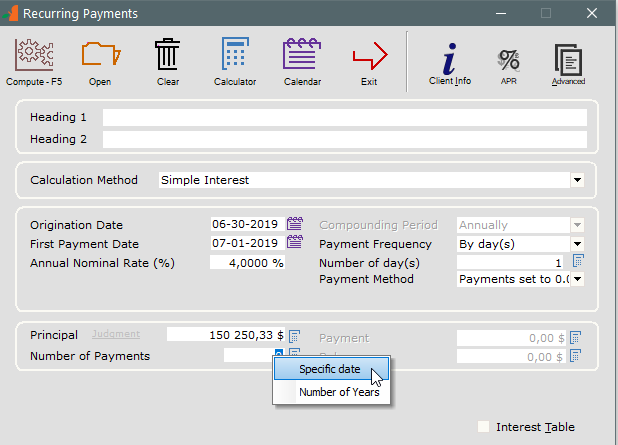We get a 333 line schedule with the daily interest for each day.

We get almost the same amount as in the calculation done with “Interest on one amount between two dates”. We are higher by 0.66 since the calculations below are done line by line and the 2 decimal point pennies leads to this slight difference.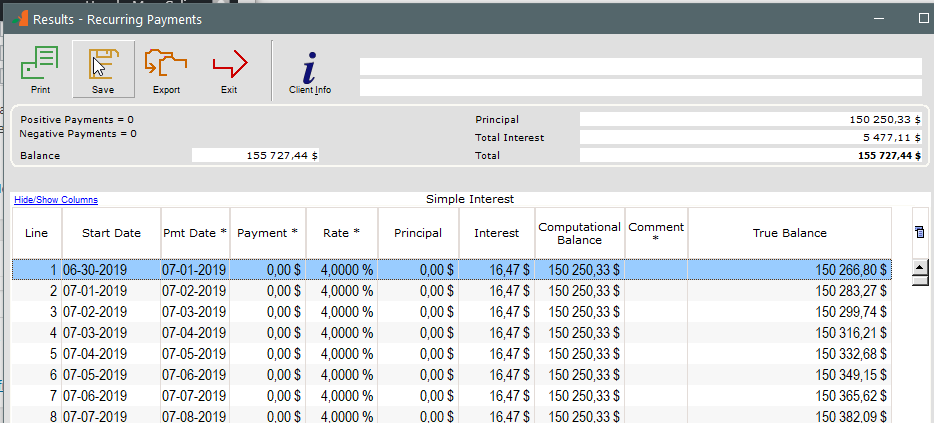—————————————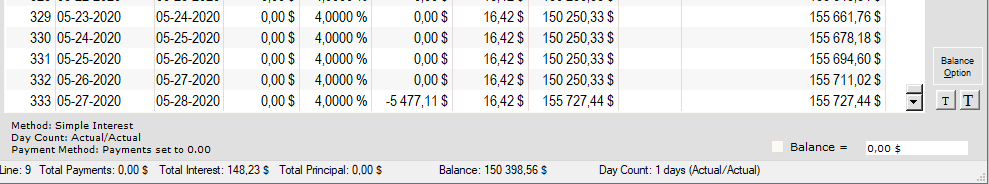In Simple interest, using the Actual/Actual Day count, the interest in 2019 is slightly higher than in leap year 2020. This also could have be done in Compound interest where the daily interest would change almost every day.

Remember the interest for the end date is excluded. So interest does not include the interest for May 28, 2020.

## How to obtain the daily interest amount (per diem) in Margill Loan Manager

Q: How to obtain the daily interest amount (per diem) in Margill Loan Manager?

A: This can be added very easily through a simple Mathematical Equation.

Go to Reports > Equation Management and click on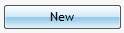1. Name the Equation
2. Select the two required fields (balance and interest rate) from the various themes on the left
3. Add the operators with the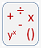button
4. Divide by 365 days with the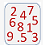button
5. Save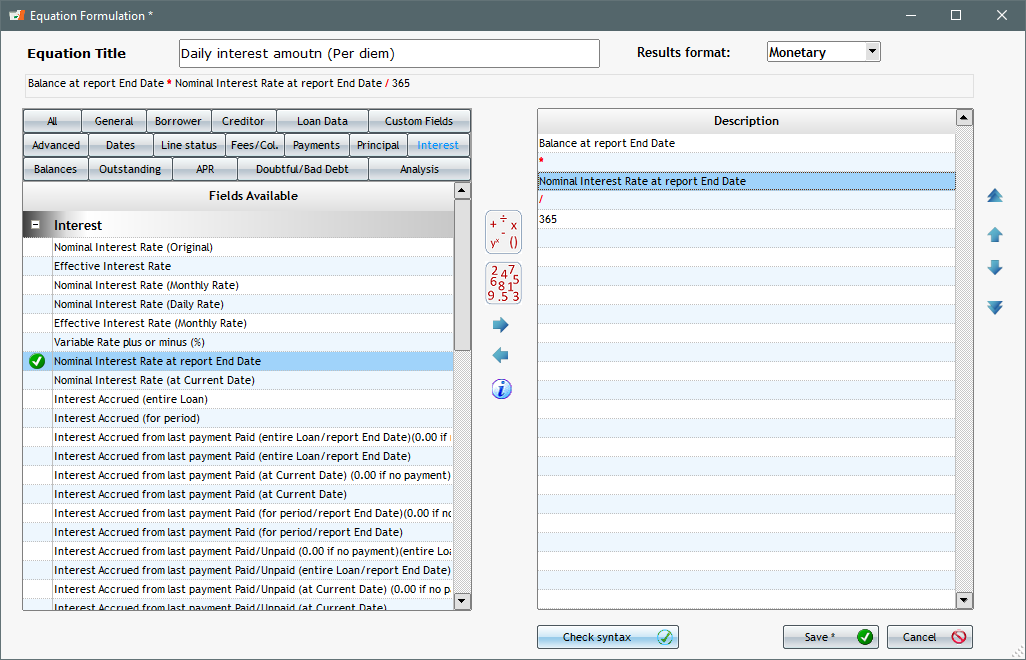You can now use this simple interest Equation in various reports picking it up in the Equations theme:

Record List Customized: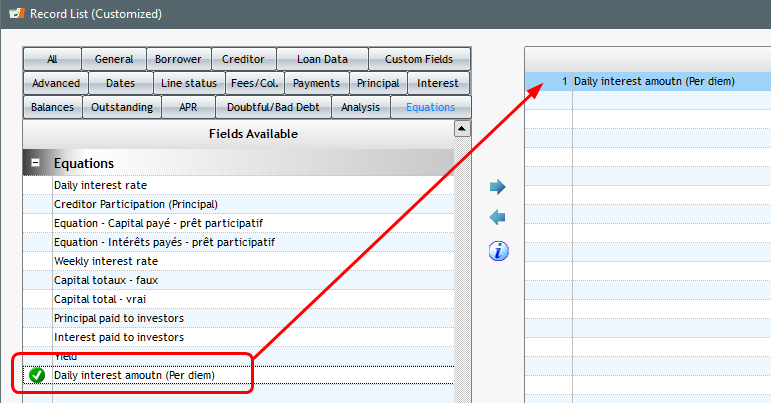Document Merge (DOCX., RTF, PDF) for your Invoices and Statements: